Friday, October 22, 2021
Home > CBSE Class 10 > Polynomials CBSE NCERT Notes Class 10 Maths Chapter 2 PDF

# Polynomials CBSE NCERT Notes Class 10 Maths Chapter 2 PDF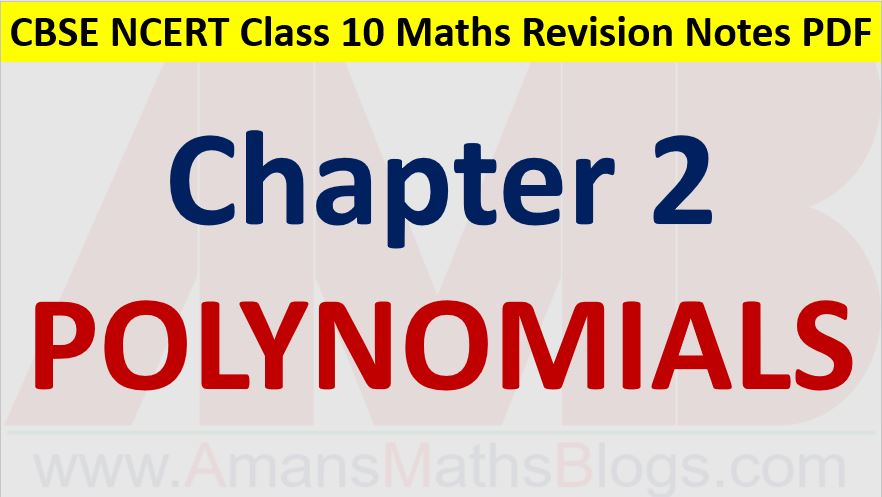Hi students, Welcome to AMBiPi (Amans Maths Blogs). In this article, you will get Polynomials CBSE NCERT Notes Class 10 Maths Chapter 2 PDF. You can download this PDF and save it in your mobile device or laptop etc.

# Polynomials CBSE NCERT Notes Class 10 Maths Chapter 1 PDF

## Polynomials

An algebraic expression p(x) of the form of p(x) = anxn + an-1xn-1 + an-2xn-2 + … + a2x2 + a1x + a0, where a0, a1, a2, …, an are real numbers and n is a non-negative integers.

The highest exponent n of the polynomial is the degree of the polynomial.

The degree of a constant polynomial p(x) = k is zero.

The degree of a zero polynomial p(x) = 0 is not defined.

The standard form of linear polynomial is p(x) = ax + b.

The standard form of quadratic polynomial is p(x) = ax2 + bx + c.

The standard form of cubic polynomial is p(x) = ax3 + bx2 + cx + d.

## Value of a Polynomial

If p(x) is a polynomial in x and k is any real number, then the value of the polynomial p(x) is obtained by replacing x by k in p(x), and is denoted by p(k).

### Class 10 Maths Chapter 2 Examples 1:

Find the value of p(x) = x2 – 3x – 4 at x = 2.

The value of given polynomial at x = 2 is p(2) = (2)2 – 3(2) – 4 = 4 – 6 – 4 = – 6.

## Geometrical Meaning of Zeroes of a Polynomial

A real number k is a zero of the polynomial p(x) if p(k) = 0.

For a linear polynomial p(x) = ax + b, a ≠ 0, the graph of y = ax + b is a straight line which intersects the x-axis at exactly one point, (-b/a, 0).

Thus, a linear polynomial p(x) = ax + b, a ≠ 0, has exactly one zero, the x-coordinate of the point where the graph of y = ax + b intersects the x-axis.

The graph of a quadratic polynomial y = p(x) = ax2 + bx + c, is a parabola.

The parabola can be upward or downward, it depends on the sign of ‘a‘ (coefficient of x2).

If the sign of ‘a‘ (coefficient of x2) in the quadratic polynomial y = ax2 + bx + c is positive, then the graph of the quadratic polynomial is an upward parabola.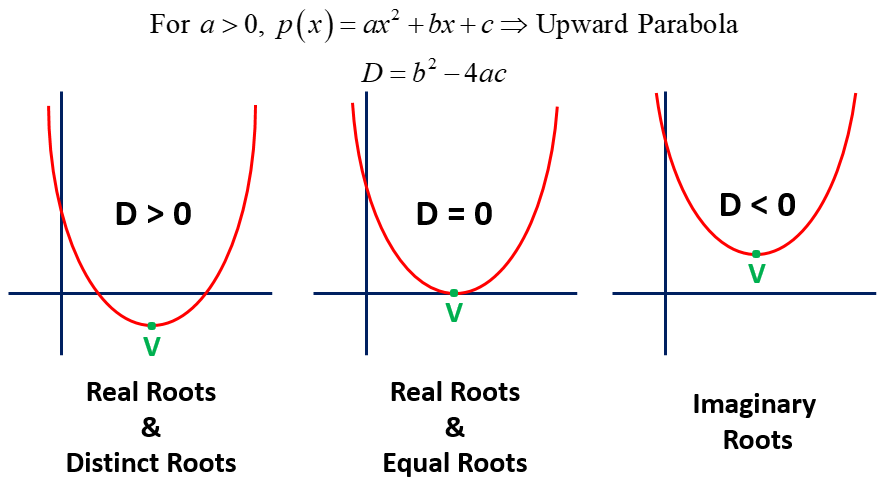If the sign of ‘a‘ (coefficient of x2) in the quadratic polynomial y = ax2 + bx + c is negative, then the graph of the quadratic polynomial is an downward parabola.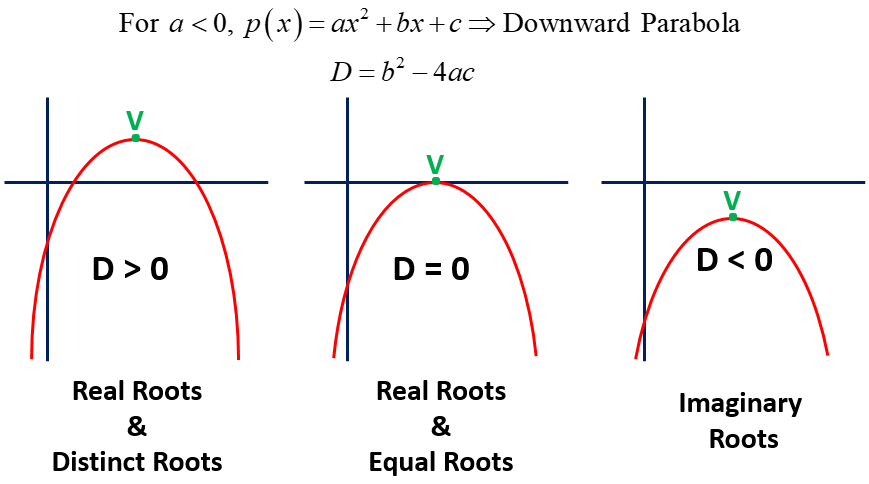If the graph of the quadratic polynomial p(x) = ax2 + bx + c intersects x-axis, then D > 0.

If the graph of the quadratic polynomial p(x) = ax2 + bx + c touches x-axis, then D = 0.

If the graph of the quadratic polynomial p(x) = ax2 + bx + c does NOT intersect x-axis, then D < 0.

For the quadratic polynomial, the zeroes of a quadratic polynomial ax2 + bx + c, a ≠ 0, are the x-coordinates of the points where the parabola representing y = ax2 + bx + c intersects the x-axis.

Thus, for a given polynomial p(x) of degree n, the graph of y = p(x) intersects the x-axis at atmost n points. Therefore, a polynomial p(x) of degree n has at most n zeroes.

### Class 10 Maths Chapter 2 Examples 2:

The graphs of y = p(x) are given below, for some polynomials p(x). Find the number of zeroes of p(x), in each case.In the graph (i), the line does not intersect x-axis. Thus, the number of zeroes of p(x) is zero.

In the graph (ii), the curve intersects x-axis at one point only. Thus, the number of zeroes of p(x) is one.

In the graph (iii), the curve intersects x-axis at three points. Thus, the number of zeroes of p(x) is three.

## Relations Between Zeroes and Coefficients of Polynomials

For quadratic polynomial p(x) = ax2 + bx + c, sum of the roots is α + β = -b/a and product of the roots is αβ = c/a.

For cubic polynomial p(x) = ax3 + bx2 + cx + d, sum of the roots is α + β + γ = -b/a and the sum of product of roots two at a time is αβ + βγ + γα = c/a and the product of the roots is αβγ = -d/a.

### Class 10 Maths Chapter 2 Examples 3:

Find the zeroes of the quadratic polynomial p(x) = x2 – 2x – 8 and verify the relationship between the zeroes and the coefficients.

p(x) = x2 – 2x – 8 = 0

⇒ x2 – 4x + 2x – 8 = 0

⇒ x(x – 4) + 2(x – 4) = 0

⇒ (x + 2)(x – 4) = 0

⇒ x = -2 or 4

α + β = (-2) + 4 = 2 = -(-2)/1 = -b/a

αβ = (-2) × 4 = -8 = -8/1 = c/a

## Formation of Polynomials

If two roots α and β are given, then the quadratic polynomial p(x) = k(x – α)(x – β) = k[x2 – (α + β)x + αβ]

If three roots α, β and γ are given, then the cubic polynomial p(x) = k(x – α)(x – β)(x – γ) = k[x3 – (α + β + γ)x2 + (αβ + βγ + γα)x – αβγ].

### Class 10 Maths Chapter 2 Examples 4:

Find a quadratic polynomial with the sum of the zeroes is 4 and the product of its zeroes is 1.

Given that α = 4 and β = 1.

Thus, the quadratic polynomial is p(x) = k[x2 – (α + β)x + αβ] = k[x2 – 5x + 4].

## Division Algorithm of Polynomials

If p(x) and g(x) are any two polynomials with g(x) ≠ 0, then there exist the polynomials q(x) and r(x) such that p(x) = g(x) × q(x) + r(x), where r(x) = 0 or degree of r(x) < degree of g(x).

### Class 10 Maths Chapter 2 Examples 5:

Divide 3x2 – x3 – 3x + 5 by x – 1 – x2, and verify the division algorithm.

The division of – x3 + 3x2 – 3x + 5 by – x2 + x – 1 is as below.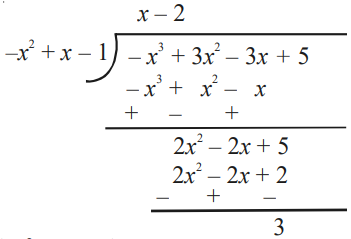Now, Divisor × Quotient + Remainder

= (–x2 + x – 1)(x – 2) + 3

= –x3 + x2 – x + 2x2 – 2x + 2 + 3

= –x3 + 3x2 – 3x + 5

= Dividend

### Class 10 Maths Chapter 2 Examples 6:

Find all the zeroes of 2x4 – 3x3 – 3x2 + 6x – 2, if you know that two of its zeroes as √2 or –√2.

Given that two zeroes are √2 and −√2. Thus, one of the factors of the given polynomial is (x + √2)(x – √2) = x2 – 2. Now, we divide the given polynomial by x2 – 2.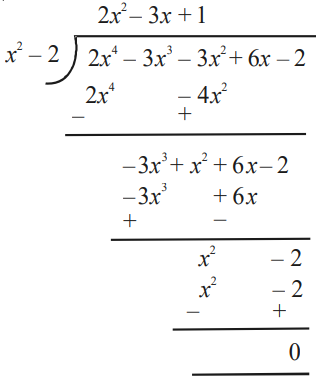2x4 – 3x3 – 3x2 + 6x – 2 = (x2 – 2)(2x2 – 3x + 1) = (x + √2)(x – √2)(2x – 1)(x – 1).

Thus, all of its zeroes are – √2, √2, 1/2, 1.

Click below to get CBSE Class 10 Maths Chapter wise Revision Notes PDF.

Chapter NoChapter name
Chapter 1 :Real Numbers CBSE Class 10 Maths Revision Notes
Chapter 2 :Polynomials CBSE Class 10 Maths Revision Notes
Chapter 3 :Pair of Linear Equations in Two Variables CBSE Class 10 Maths Revision Notes
Chapter 4 :Quadratic Equations CBSE Class 10 Maths Revision Notes
Chapter 5 :Arithmetic Progression CBSE Class 10 Maths Revision Notes
Chapter 6 :Triangles CBSE Class 10 Maths Revision Notes
Chapter 7 :Coordinate Geometry CBSE Class 10 Maths Revision Notes
Chapter 8 :Introduction to Trigonometry CBSE Class 10 Maths Revision Notes
Chapter 9 :Some Applications of Trigonometry CBSE Class 10 Maths Revision Notes
Chapter 10 :Circles CBSE Class 10 Maths Revision Notes
Chapter 11 :Constructions CBSE Class 10 Maths Revision Notes
Chapter 12 :Area Related to Circles CBSE Class 10 Maths Revision Notes
Chapter 13 :Surface Areas & Volumes CBSE Class 10 Maths Revision Notes
Chapter 14 :Statistics CBSE Class 10 Maths Revision Notes
Chapter 15 :Probability CBSE Class 10 Maths Revision Notes
AMBiPi
error: Content is protected !!